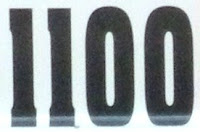## Tuesday, June 7, 2011

### 1100

1100 = 22 x 52 x 11.

1100 has a base 2 (binary) representation that ends in the digits 1100: 10001001100. 1100 is 3131 in base 7. It is 778 in base 12, 668 in base 13, and 588 in base 14.

1100 is the sum of two palindromic primes in three ways: 1100 = 181 + 919 = 313 + 787 = 373 + 727.

1100 is the sum of a square and a cube: 1100 = 103 + 102. It is the sum of two distinct powers of 10.

1100 is the sum of eight positive fifth powers: 1100 = 35 + 35 + 35 + 35 + 25 + 25 + 25 + 25.1100 AD is an online multiplayer strategy games set in medieval times.